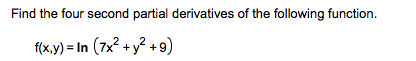# Find the four second partial derivatives of the following functionf(xy)-In (7x2 + y2 +9)

Question
1 viewshelp_outlineImage TranscriptioncloseFind the four second partial derivatives of the following function f(xy)-In (7x2 + y2 +9) fullscreen
check_circle

Step 1

To calculate all the second order partial derivatives of the given function of two variables

Step 2

Partial differentiation of (any) function with respect to the variable x (y) is performed keeping the variable y (x) constant. There are four second order partial derivatives for a function of two variables.

Step 3

### Want to see the full answer?

See Solution

#### Want to see this answer and more?

Solutions are written by subject experts who are available 24/7. Questions are typically answered within 1 hour.*

See Solution
*Response times may vary by subject and question.
Tagged in

### Calculus